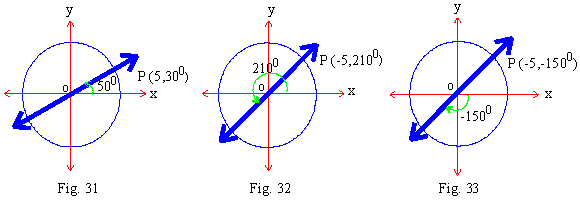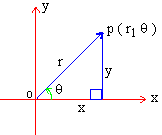Support the Monkey! Tell All your Friends and TeachersHome MonkeyNotes Printable Notes Digital Library Study Guides Study Smart Parents Tips College Planning Test Prep Fun Zone Help / FAQ How to Cite New Title Request

 7.5 Polar Co-ordinates We know how to represent points and curves in Cartesian coordinates. Another system for such representation is the Polar System. In this system, the position of a point P on a plane is known, if we are given (i) Its distance 'r' from a fixed point O (ii) The inclination q of OP to OA, a fixed line through O. Here the fixed point is called the pole, or origin. The fixed line OA (which is usually situated horizontally) is called the polar axis. 'r' is called the 'radius vector' of P and 'q' is called the 'vectorial angle'. If the angle is measured counter clock-wise sense, it is positive or if it is measured in clockwise sense, then it is negative. Note that point P if on the terminal side of angle q then r is positive. If P is on the opposite side of the pole then r is negative. The two 'r' and 'q', are now called the polar coordinates of P and written as an ordered pair (r,q). The location of a point can be named in many different pairs of polar coordinates. Following figures illustrate three different sets of Polar Coordinates of point P (5, 300).Click here to enlarge For changing from Cartesian to Polar coordinates, or vice versa, we take origin as pole. The x-axis as the initial line or the polar axis.Then x = r cos q,     y = r sin q ........(i) are the formulas, for converting from Cartesian to Polar from (i) x2 + y2 = r2   and   q = tan-1 (y/x) .....(ii) We use (ii) for the conversion from Polar to Cartesian Co-ordinates. Index Chapter 8
 Search: All Products Books Popular Music Classical Music Video DVD Toys & Games Electronics Software Tools & Hardware Outdoor Living Kitchen & Housewares Camera & Photo Cell Phones Keywords: